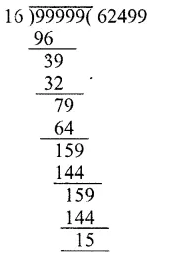# RS Aggarwal Solutions Class 6 Chapter 3 Whole Numbers Exercise 3F

In this chapter, we provide RS Aggarwal Solutions for Class 6 Chapter 3 Whole Numbers Exercise 3F for English medium students, Which will very helpful for every student in their exams. Students can download the latest RS Aggarwal Solutions for Class 6 Chapter 3 Whole Numbers Exercise 3F pdf, free RS Aggarwal Solutions Class 6 Chapter 3 Whole Numbers Exercise 3F book pdf download. Now you will get step by step solution to each question.

### RS Aggarwal Class 6 Chapter 3 Whole Numbers Ex 3F Download PDF

Question 1.
Solution:
The smallest whole number is 0 (b)

Question 2.
Solution:
The least 4-digit number = 1000
On dividing 1000 by 9, we get
Remainder = 1Least 4-digit number which is
Divisible by 9 = 1000 – 1 + 9
= 1000 + 8
= 1008 (d)

Question 3.
Solution:
The largest 6-digit number = 999999
On dividing by 16, we get
Remainder =15The greatest 6-digit number divisible by 16
= 999999 – 15
= 999984 (c)

Question 4.
Solution:
On dividing 10004 by 12, we get remainder = 88 is to be subtracted from 10004 (c)

Question 5.
Solution:
On dividing 10056 by 23 We get remainder =12The least number to be added = 23 – 5
= 18 (b)

Question 6.
Solution:
On dividing 457 by 11
We get remainder = 6Which is greater than half of 11
The number nearest to 457 which is divisible 11 will be = 457 – 6 + 11
= 457 + 5
= 462 (d)

Question 7.
Solution:
Whole number between 1018 and 1203 are 1019 to 1202 are 1202 – 1018
= 184 (c)

Question 8.
Solution:
Divisor = 46
Quotient =11
Remainder =15
Number = Divisor x Quotient + Remainder
= 46 x 11 + 15
= 506 + 15
= 521 (b)

Question 9.
Solution:
Dividend = 199
Quotient =16
Remainder = 7
Divisor = 199−716 = 19216
= 12 (c)

Question 10.
Solution:
7589 – ? = 3434
Required number = 7589 – 3434
= 4155 (c)

Question 11.
Solution:
587 x 99 = 587 x (100 – 1)
= 587 x 100 – 587 x 1
= 58700 – 587
= 58113 (c)

Question 12.
Solution:
4 x 538 x 25 = 538 x 4 x 25
= 538 x 100
= 53800 (c)

Question 13.
Solution:
24679 x 92 + 24679 x 8
= 24679 x (92 + 8)
= 24679 x 100
= 2467900 (c)

Question 14.
Solution:
1625 x 1625 – 1625 x 625
= 1625 (1625 – 625)
= 1625 x 1000
= 1625000 (a)

Question 15.
Solution:
1568 x 185 – 1568 x 85
= 1568 (185 – 85)
= 1568 x 100
= 156800 (c)

Question 16.
Solution:
888 + 111 + 555 = 111 x ?
= 11 (8 + 7 + 5)
= 111 x 20 (c)

Question 17.
Solution:
The sum of two odd number is an even number (b)

Question 18.
Solution:
The product of two odd numbers is an odd number (a)

Question 19.
Solution:
If a is a whole number such that
a + a = a, then a = 0
as 0 + 0 = 0
None of these (d)

Question 20.
Solution:
The predecessor of 10000 is 10000 – 1
= 9999 (b)

Question 21.
Solution:
The successor of 1001 is 1001 + 1
= 1002 (b)

Question 22.
Solution:
The smallest even whole number is 2 (b)

All Chapter RS Aggarwal Solutions For Class 6 Maths

—————————————————————————–

All Subject NCERT Exemplar Problems Solutions For Class 6

All Subject NCERT Solutions For Class 6

*************************************************

I think you got complete solutions for this chapter. If You have any queries regarding this chapter, please comment on the below section our subject teacher will answer you. We tried our best to give complete solutions so you got good marks in your exam.

If these solutions have helped you, you can also share rsaggarwalsolutions.in to your friends.